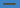# How to write palindrome program using JavaScript

Home » javascript » How to write palindrome program using JavaScriptIn this tutorial, we are going to learn how to implement palindrome program in JavaScript.

## What is Palindrome?

A palindrome is a word or number or phrase which can be read same as backward and forward.

for example: mom, LoL, 11011, 10201 or “A man, a plan, a canal, Panama!“.

Let’s write our function to check is give value is palindrome or not.

Note: we are using regular expression `[\W_]` to remove the non alpha numeric characters such as `(\$ , % , @, !)` . These are alpha (A-Z,a-z) numeric(0-9) characters.

``````function palindrome(str) {

// remove the non alpha numeric characters and lower case the string.

let lowercase = str.replace(/[\W_]/gi, '').toLowerCase()

// reverse the lower case string

let stringreverse = lowercase.split('').reverse().join('');

// compare both lowercase and stringreverse

if (lowercase === stringreverse) {
return true
} else {
return false
}
}
console.log(palindrome("A man, a plan, a canal, Panama!"));
// true``````
• In the above code first, we removed the all non-alphanumeric characters from the string by using regex([/W_]) inside the replace method then we lowercased the string.
• Next step, we reversed the lower case string. (because we need to read the same as backward).
• The final step, we compared both strings and returned true if its palindrome otherwise we return false.

We can also refactor our code in two lines instead of using variables.

``````function palindrome(str){

let lowercase =  str.replace(/[\W_]/gi,'').toLowerCase()

return lowercase  === [...lowercase].reverse().join('')
}``````